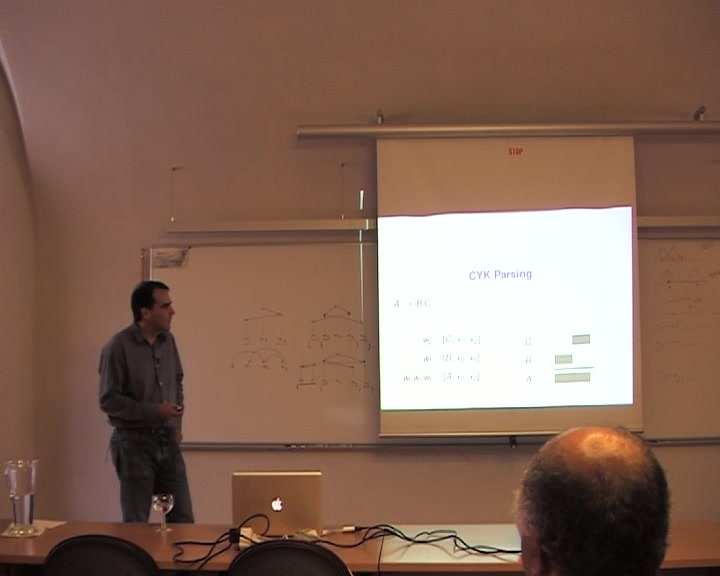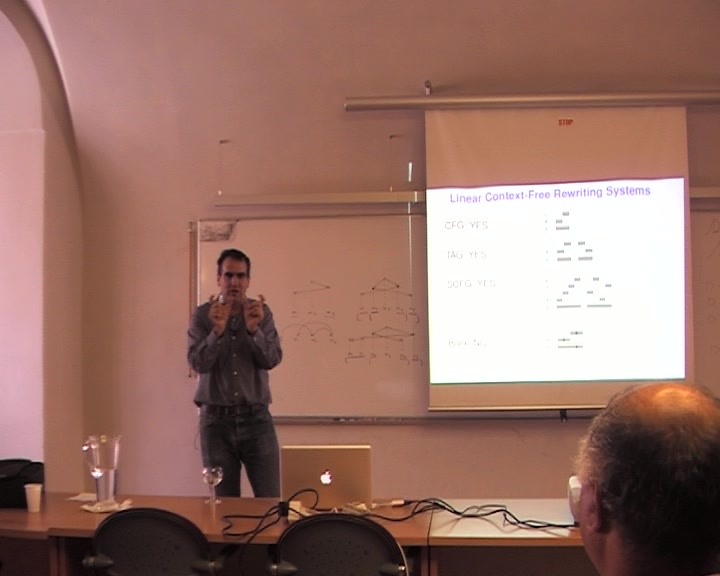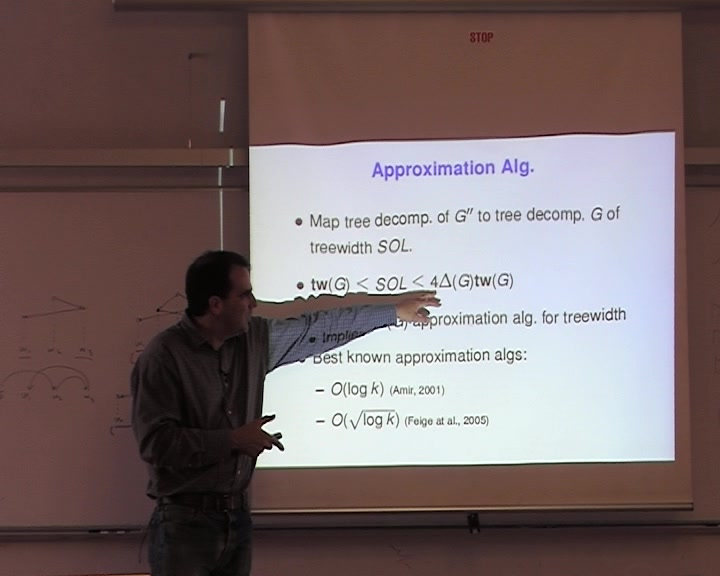### Grammar Factorization by Tree Decomposition

Speaker:
Dan Gildea
Abstract:
We describe the application of the graph-theoretic property known as treewidth to the problem of finding efficient parsing algorithms. This method, similar to the junction tree algorithm used in graphical models for machine learning, allows automatic discovery of efficient algorithms such as the O(n^4) algorithm for bilexical grammars of Eisner and Satta (1999). We examine the complexity of applying this method to parsing algorithms for general Linear Context-Free Rewriting Systems (LCFRS). We show that any polynomial-time algorithm for this problem would imply an improved approximation algorithm for the well-studied treewidth problem on general graphs.
Length:
00:00:00
Date:
30/07/2014
views: 1045

Images:Attachments: (video, slides, etc.)
39 MB